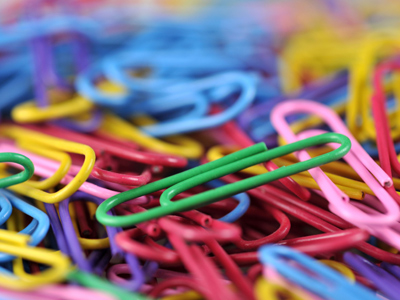You would use grams to measure the weight of a paper clip.

# Measures (Year 6)

In KS2 Year Six, children should be quite comfortable when dealing with measures in Maths. By now they should be well aware of the metric measures (mm, cm, m, km, g, kg, tonnes, ml, cl and l) and know some of the imperial measures (pint, gallon, mile etc.). They should also be able to convert smaller and larger units and combine the two (eg. 2.8 m = 280 cm = 2m 80cm). They will now be introduced to the imperial units of weight – ounces, pounds and tons, and how they relate to grams, kilograms and tonnes.

Tons, stones, pounds and ounces are all types of measures. They are all used to measure weight, but nowadays tonnes, kilograms and grams are more common. Babies used to be weighed in pounds and ounces when they were first born. These days, they are weighed in grams. Are you able to change from pounds and ounces to grams quickly and easily?

Which weight is highest - 1,000 kilograms or 1 ton? Find out in this measures quiz.

1.
100 centilitres is equal to?
1 litre
10 litres
100 litres
1,000 litres
We can shorten centilitre to cl
2.
Which unit would you use to measure the weight of a paper clip?
kg
ml
cm
grams
Some paperclips would probably weigh even less than a gram!
3.
What is 4.219km in metres?
42.19m
421.9m
4,219m
42,190m
To convert kilometres to metres, times by 1,000
4.
How do we write 375ml in litres?
0.375 l
3.75 l
37.5 l
375 l
To convert millilitres to litres, divide by 1,000
5.
How many ml in 1 centilitre?
1ml
10ml
100ml
1,000ml
1 litre = 100cl = 1,000ml
6.
How many miles in 8 km?
0.5 mile
5 miles
50 miles
500 miles
To convert km to miles divide by 8 and then times by 5.
To convert miles to km divide by 5 and then times by 8
7.
Approximately how many grams are equal to 1 ounce?
0.3g
3g
30g
300g
1oz (1 ounce) = 28.3g
8.
How many kg in one tonne?
10kg
100kg
1,000kg
10,000kg
A non-metric ton is equal to 1,016kg - slightly heavier than a metric tonne
9.
Which unit would you use to measure the distance between the Moon and Earth?
cm
metres
mm
miles
Kilometres could also be used
10.
Roughly how many pounds in 1kg?
1 pound
2 pounds
2.2 pounds
2.5 pounds
1lb (1 pound) = 454g